# Solution assignment 09 Linear functions and graphs

### Assignment 9

Given the following two linear equations with two unknown variablesen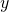: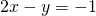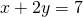1. The equations represent lines. Calculate their slopes.
2. How can you calculate the coordinates of the intersection point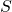of both lines?

### Solution

1. The first equation can be written as: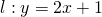and has the slope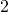.
The second equation can be written as: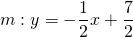and has the slope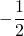.
We can calculate the-coordinate of the intersection pointfrom: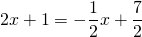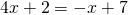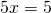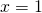and so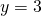The coordinates ofare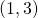.

An alternative calculation.
We could have calculated this result directly from the two previous equations:Just multiply the second equation with:and subtract it from the first equation. Then we get: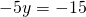and so.